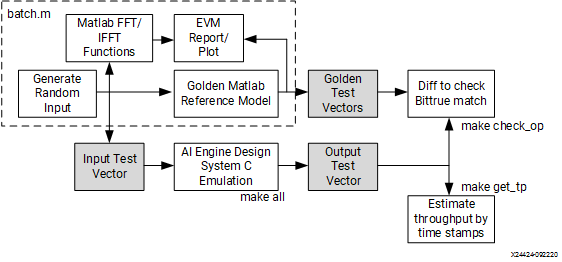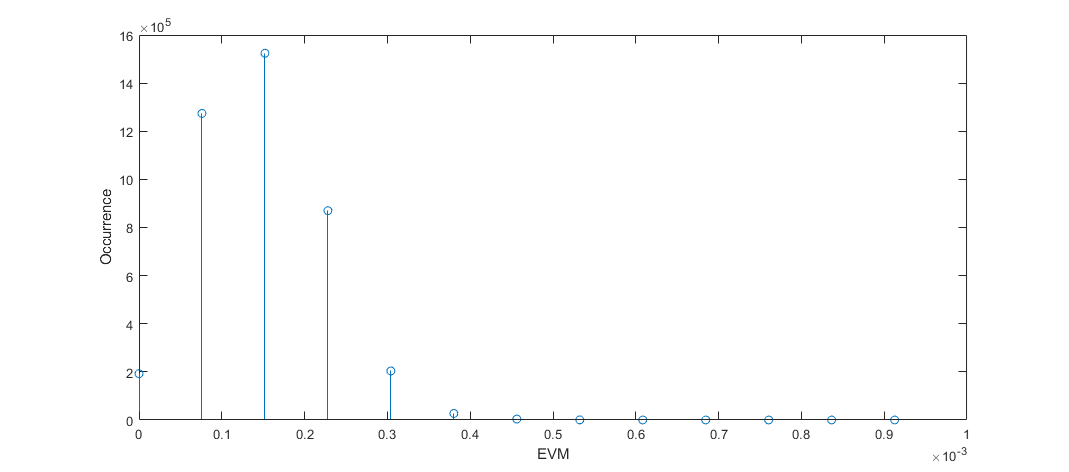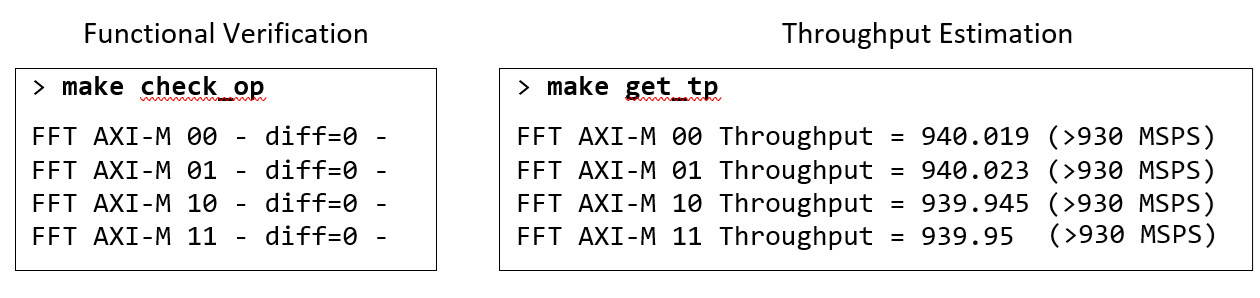# Design Validation

## Block-by-Block Configurable Fast Fourier Transform Implementation on AI Engine (XAPP1356)

Document ID
XAPP1356
Release Date
2021-01-11
Revision
1.0 English

AI Engine designs can be simulated for functional verification and throughput validation before integration with programmable logic. The following figure shows the validation workflow of the FFT reference design.

Figure 1. AI Engine Design Validation WorkflowRandom input test vectors are generated by a MATLAB® script and golden test data are computed by the MATLAB reference model. The computation results of the MATLAB bit-true model are compared to those of MATLAB double-precision fft() and ifft() functions and the normalized error-vector magnitudes (EVM) are reported. The following figure is the EVM histogram calculated from 1000 random input data blocks. It is also shown that the maximum EVM is 0.08%, and the root mean square error is 0.02%.

Figure 2. EVM Histogram of MATLAB Bit-true Reference ModelAI Engine designs are compiled and tested in a System C simulation environment (AI Engine simulator) using the input test vectors generated by MATLAB. The simulation results are stored in data files that record the output samples along with their time stamps. The time duration from the first output sample to the last can be measured by the time stamps, and the number of output samples can be counted. Their quotient gives an estimate of throughput. The FFT reference design has four output AXI streams. A Makefile is included in the design to compare all four outputs with the reference test vectors. In the following figure, the left column shows a bit-true match with the reference output for the FFT design. The Makefile also estimates the throughput from all output files. The right side of the figure shows that all four output AXI streams achieve the target throughput of 925 MSPS with some margin. The total throughput of this design of 10 AI Engines is measured at 3.76 GSPS.

Figure 3. Example Design Validation Result